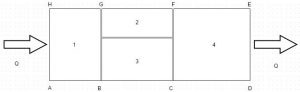# E-PolyLearning

## Discussion Forum

 Que. Find the heat flow rate through the composite wall as shown in figure. Assume one dimensional flow and take k 1 = 150 W/m degree k 2 = 30 W/m degree k 3 = 65 W/m degree k 4 = 50 W/m degree AB = 3 cm, BC = 8 cm and CD = 5 cm. The distance between middle horizontal line from the top is 3 cm and from the bottom is 7 cma. 1173.88 W b. 1273.88 W c. 1373.88 W d. 1473.88 W